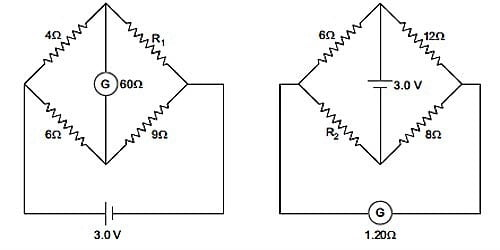# Explain Current Sensitivity of a Galvanometer

The current sensitivity of a galvanometer is defined as the deflection produced when unit current passes through the galvanometer. A galvanometer is said to be sensitive if it produces large deflection for a small current.

In a galvanometer, I = (C/nBA) x θ

∴ Current sensitivity θ/I = nBA/C … (1)

The current sensitivity of a galvanometer can be increased by

(i) increasing the number of turns

(ii) increasing the magnetic induction

(iii) increasing the area of the coil

(iv) decreasing the couple per unit twist of the suspension wire.

This explains why phosphor-bronze wire is used as the suspension wire which has a small couple per unit twist.

Current sensitivity of a galvanometer is defined as the deflection produced in the galvanometer when a unit current flows through it.

IS = θ/I = nBA/c

Where n is no of turns in the coil of the galvanometer, B is Magnetic field around the coil, A is Area of the coil and c is restoring torque per unit twist.

The sensitivity of Moving Coil Galvanometer

Moving coil galvanometer is an electromagnetic device that can measure small values of current. The sensitivity of a Moving Coil Galvanometer is defined as the ratio of the change in deflection of the galvanometer to the change in current. Therefore we write, Sensitivity = dθ/di. If a galvanometer gives a bigger deflection for a little current it is said to be sensitive. The current in Moving Coil galvanometer is: I = (C/nBA) × θ

The sensitivity of Moving Coil Galvanometer increases by:

• Increasing the no. of turns and the area of the coil,
• Increasing the magnetic induction and
• Decreasing the couple per unit twist of the suspension fiber.